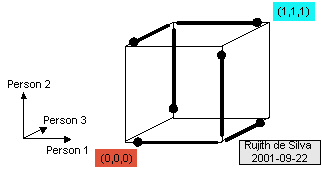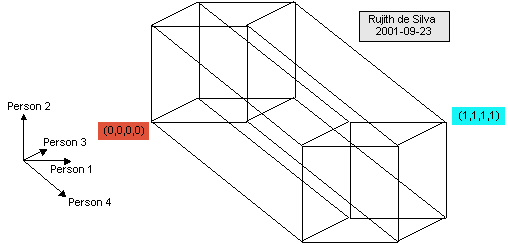# Three Crowns Paradox: Lollipops in Hyperspace

A fascinating paradox was discovered by Todd Ebert in 1998 (see the links at the end). Its solutions are well known, but this document describes an interesting approach to visualizing the paradox and its solutions that will hopefully help in intuitively grasping the paradox.

The paradox is described below, then it is re-cast as a search problem in hyper-space. The solution and its variants are shown for the three-player case. Then the four-player case is shown, and the approaches to its solution are discussed.

## Description of the Paradox

Three players enter a room and a red or blue crown is placed on each person's head. The colour of each crown is determined by a coin toss, with the outcome of one coin toss having no effect on the others. Each person can see the other players' crowns but not his own.

No communication of any sort is allowed, except for an initial strategy session before the game begins. Once they have had a chance to look at the other crowns, each player must simultaneously guess the colour of their own crown or pass. The group wins if at least one player guesses correctly and no player guesses incorrectly.

If a player chooses to guess, then there's a 1/2 chance that his guess will be wrong, as he has no information about the colour of his own crown. The paradox is that the team can collectively win more than 1/2 the time, even though each individual's guess is correct only 1/2 the time.

If you wish to solve the paradox, stop now and read the rest later!

## Representing the Paradox

How can this problem be represented? Each of the three players may have a red or a blue crown. Hence there are 2 x 2 x 2 = 8 possibilities for the crowns they don. These may be conveniently be represented by a 2 x 2 x 2 cube, as shown below.Each of the three axes corresponds to one of the three people. Red has been arbitrarily marked as 0, and blue as 1. Then each vertex is uniquely identified by a tuple such as (0,1,1) - this denotes Player 1 having a red crown, and Players 2 and 3 having blue crowns.

Each player's task is to guess the vertex corresponding to the actual colours, while being unaware of his own colour. This corresponds to being unable to distinguish between vertices that are joined by an edge parallel to that person's axis. For example, Person 1 cannot distinguish between the vertices (0,0,0) and (1,0,0), the two bottom-most vertices, because the edge joining them is parallel to the axis for Player 1. So he has no basis for preferring one of those vertices over the other.

Hence the strategy for a given player can be represented by lollipops whose edges are parallel to that player's axis. For example, suppose that Player 1 can see that Players 2 & 3 have red crowns. So he knows that the possible vertices are (0,0,0) and (1,0,0). He is forced to decide whether to guess red, guess blue, or pass. A guess of blue by Player 1 would be denoted by a lollipop as follows:The lollipop's stick denotes the vertices that the user had to choose between, and the head of the lollipop denotes the vertex that he decided to choose.

If Player 1 decides to pass, that is denoted by the absence of a lollipop along the relevant edge.

So the search for a strategy can be re-cast as a search for good placements of these lollipops. This is arguably a better search-space for humans, particularly in the three-player situation, as we have excellent mental machinery for manipulating 3-dimensional entities.

What rules govern the placement of the lollipops?

1. Each edge has at most one lollipop.
2. A vertex is considered "won" if it adjoins the head-end of at least one lollipop, and does not adjoin the stick-end of any lollipop.
3. The value of a strategy is the number of "won" vertices, divided by the total number of vertices. This gives the probability of winning the game with that strategy.

A straightforward but important corollary is that if a vertex adjoins the stick-end of a lollipop, then that vertex is already lost, and there is no further penalty if further lollipops are placed with their stick-ends adjoining that vertex.

This leads to the following strategy: pick one vertex and "sacrifice" it. Then place as many lollipops as possible with their stick-ends adjoining the "sacrificed" vertex. Repeat until all the vertices have been covered.

## Optimal Strategies for Three Players

Following the above strategy in the 2 x 2 x 2 cube for three players leads to the following strategy:Two vertices were "sacrificed", and three lollipops placed at each of the two sacrificed vertices, thereby securing six vertices as "won." Thus the value of the strategy (or, equivalently, the probability of winning via this strategy) is equal to 6 / 8 = 3/4.

Note that any given guess has a 1/2 chance of being incorrect. So how does the overall strategy surpass the probability of the individual guesses? This is because when a guess by a player is wrong, then the guesses by the other two players are also wrong, denoted by the profusion of lollipops sticking out of the "sacrificed" vertices.

## Guarding against a Myth of Probability

The three-player strategy described above can be stated succinctly as "If the other two players have the same coloured crowns, then guess that mine is the opposite colour, otherwise pass."

This is a completely accurate strategy, and is probably the easiest to describe of the several optimal strategies. However, there is a common myth regarding probability, viz., if a coin comes up heads several times, then it is likelier to come up tails the next time. Or that if a roulette wheel has yielded red several times, then it will yield black the next time. The strategy described above is suspiciously like an endorsement of this myth of probability, viz., that if the other two players have the same coloured crowns, then the third player is more likely to have a opposite coloured crown.

That belief is completely unfounded, and is not the cause of the strategy's success. If two players have red crowns, then the chance that the third player has a blue crown is still precisely and exactly 1/2.

The true source of the strategy's success can be seen by examining other optimal strategies, reached by placing the lollipops differently. For example, a simple rotation of the lollipops gives the strategy:In this case, Player 1's strategy is "If the other two players have the same coloured crowns, then guess that mine is the same colour" - the opposite of what the probability myth would recommend! The strategy for Players 2 and 3 is "If the other two players have differently coloured crowns, then guess that mine is the same colour as Player 1's."

## Lollipops in the Fourth Dimension

Any number of players can take part in the guessing game. The basic strategy remains the same, and the search-space can be re-cast as the placement of lollipops in a hyper-cube. However, the dimensions of the hyper-cube must equal the number of players, and our mental machinery finds it more difficult to intuitively grasp the problem as the number of dimensions increases. The four-player version is barely graspable:This shows the 2 x 2 x 2 x 2 four-dimensional hyper-cube for four players as well as possible in two dimensions. But the rules for the placement of the lollipops are exactly the same. So how well can we do in this case? One strategy is to basically ignore the fourth player, who will always pass. The other three players will play the same strategy as in the three-player version. This corresponds to the following placement of lollipops:Four vertices are sacrificed, and twelve won. The probability of winning with this strategy is 12 / 16 = 3/4, the same as with the three-player game.

Can we do better? First of all, how many vertices must be sacrificed to win the remainder? At each sacrificed vertex, a lollipop can be placed (stick-end adjoining) along each of the four axes. Thus, a maximum of four vertices can be won for each sacrificed vertex. If three vertices are sacrificed, then 3 x 4 = 12 can be won. This covers 3 + 12 = 15 vertices. That leaves a single remaining vertex, and there is no way to win it, as there are no more vertices left to sacrifice!

In practice, there is no way to place the lollipops to win 12 vertices by just sacrificing three of them. This becomes evident upon attempting to place the lollipops. The best that can be done leads to solutions such as:In this strategy, two sacrificed vertices have their full complement of lollipops sticking out along all four axes. But there is no way to cover the remaining vertices by sacrificing just one vertex. Instead, two vertices are sacrificed, and two lollipops are placed adjoining each of them.

However, as speculated by Ryan Leigland, an arbitrarily high probability of winning can be achieved by increasing the number of players. In particular, for any positive integer n, if there are 2n - 1 players, then the probability of losing is 1/2n. When n is 1, then a single player has 1/2 chance of losing; when n is 2, then three players have 1/4 chance of losing; when n is 3, seven players have 1/8 chance of losing; and so on. This can be achieved by constructing perfect error-correcting Hamming codes for n bits such that each bit-pattern has a Hamming distance of 3 from its nearest neighbours. Then the sacrificed vertices correspond to the accepted bit-patterns in the error-correcting code.

## Conclusion

Placing lollipops in hyper-space is hopefully a good way to intuitively grasp how this paradox works, and may help in finding solutions. This system is described more formally below.

## Formalities

A lollipop can be conveniently denoted using a notation such as (1,0,0), where the bold number indicates the person making the guess, viz., that Player 1 decides to guess blue if the other two players have red crowns. A strategy is a collection of such lollipops. For example, the simplest optimal strategy for three players is:

 ( 1,0,0) ( 0,1,1) (0, 1,0) (1, 0,1) (0,0, 1) (1,1, 0)

The key to placing the lollipops is finding the vertices to sacrifice. This corresponds to finding as many vertices as possible whose Hamming distance is 3.

## Links

The paradox was originally described at: www.ics.uci.edu/~ebert/teaching/spring2001/ics151/puzzles.html, but now that page has disappeared. There's a passing reference at rangevoting.org/PuzzlePage.html#p3. If you know any other source, I'd like to know. Thanks!

Rujith de Silva
Created 2001-09-25; modified 2006-06-02.